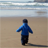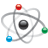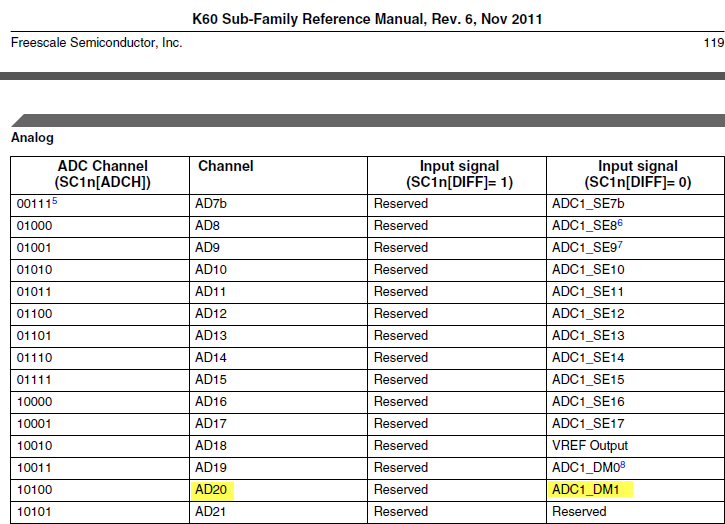cancel
Showing results for
Did you mean:
SOLVED

839 ViewsSenior Contributor I

Hi All,

For the adc demo project, when I tried it, I adjusted the potentiometer and used a voltage meter to measure the voltage between pin2 and pin3  of potentiometer.

When the voltage between pin2 and pin3 are zero, the adc reading is 32267. When the voltage between pin2 and pin3 is 3.28v, the adc reading is 15 or sometimes 65530.

Thank you.

-Daniel

1 Solution
308 ViewsContributor III

The 16 bit result is a signed 2's complement. The most significant bit is the sign. That means that positive results go from 0x0000 to 0x7FFF (= 32767). And negative results start at 0xFFFF (=-1) up to 0x8000 (=-32768). Note how the direction of negative results is reversed.

7 Replies
308 ViewsContributor III

I am looking at the Schematics of the TWRK60N512 RevD. The potentiometer pin 2 is connected to ADC1_DM1. The second differential input of the ADC  ADC1_DP1 is pulled high to 3.3V on one of the 20pin connectors.

If your ADC software is measuring differential than indeed  3.28V will equal a reading close to zero and when pin2 is at 0V than the ADC measures the pullup voltage of 3.3V which corresponds to about 32267.

So everything is ok. The potentiometer is just connected in a strange way.

On a side note, beware that the potentiometer is a 5K resistor and the input resistance of the ADC is also 5K. This is not ideal since for a precise measurement the ADC should have a much higher resistance. So dont expect precise results from this setup.

308 ViewsNXP Employee

The potentiometer signal is connected to the differential ADC input pin ADC1_DM1.  But you can also setup the ADC to do single-ended conversions on some of the differential pins.  If you want to do the single-ended conversion with the MQX example, you can change the ADC channel used in the structure for the ADC driver from ADC1_SOURCE_ADPM1 to ADC1_SOURCE_AD20 in adc_demo.c.  Now if you test the example, you will see the converted value scale from 0 to 65535.

The Chip Configuration Chapter 3 in the reference manual contains the channels to use for the ADC.  If you want single-ended conversions, look under the column with the DIFF bit = 0.  Below is the table showing why ADC1_SOURCE_AD20 channel should be used for a single-ended measurement of the ADC1_DM1 signal.308 ViewsContributor II

Thanks, looked for this information for like 2 hrs until I found this thread. Should have just done a search in the PDF

308 ViewsSenior Contributor I

Derek,

Thank you very much.

-Daniel

308 ViewsSenior Contributor I

Thank you.

I am confused about the reading 32267. Because I am using ADC 16bits resolution, the reading should be around 65536 when pin2 is 0. Is it correct? or it divides the ADC result to positive and negative, so the largest positive number is 32767 for 16 bits.

Thank you again.

-Daniel

309 ViewsContributor III

The 16 bit result is a signed 2's complement. The most significant bit is the sign. That means that positive results go from 0x0000 to 0x7FFF (= 32767). And negative results start at 0xFFFF (=-1) up to 0x8000 (=-32768). Note how the direction of negative results is reversed.

308 ViewsSenior Contributor I

Thank you very much. It helps a lot.